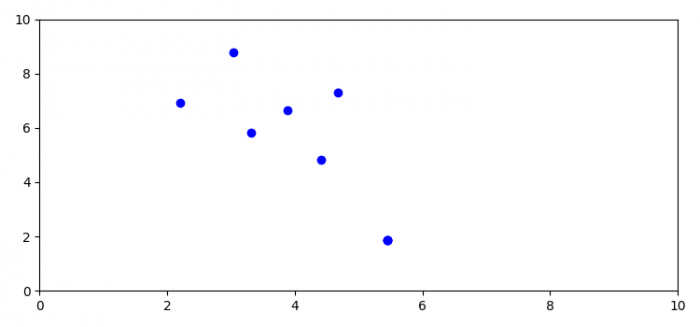# How can I pass parameters to on_key in fig.canvas.mpl_connect('key_press_event',on_key)?

MatplotlibPythonData Visualization

To pass parameters to on_key in fig.canvas.mpl_connect('key_press_event', on_key), we can take the following steps −

• Set the figure size and adjust the padding between and around the subplots.
• Create a figure and a set of subplots.
• Set x and y scale of the axes.
• Bind the function to the event.
• To display the figure, use show() method.

## Example

import matplotlib.pyplot as plt
plt.rcParams["figure.figsize"] = [7.50, 3.50]
plt.rcParams["figure.autolayout"] = True

fig, ax = plt.subplots()
ax.set_xlim(0, 10)
ax.set_ylim(0, 10)

def onkey(event):
if event.key.isalpha():
if event.xdata is not None and event.ydata is not None:
ax.plot(event.xdata, event.ydata, 'bo-')
fig.canvas.draw()

cid2 = fig.canvas.mpl_connect('key_press_event', onkey)
plt.show()

## Output